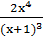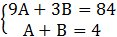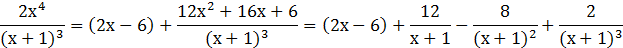### Sample Problem

Write the partial fraction decomposition of.

#### Solution

First, use long division to make the expression into a proper rational expression.Multiply both sides by (x+1)3 [the lowest common denominator]

12x2+16x+6=A(x+1)2+B(x+1)+C

Let x=-1,

12x2+16x+6=A(x+1)2+B(x+1)+C

12(-1)2+16(-1)+6=A(-1+1)2+B(-1+1)+C

12-16+6=C

2=C

Let x=0, and plug in C=2

12x2+16x+6=A(x+1)2+B(x+1)+C

12(0)2+16(0)+6=A(0+1)2+B(0+1)+(2)

6=A+B+2

4=A+B

Let x=2, plug in C=2

12x2+16x+6=A(x+1)2+B(x+1)+C

12(2)2+16(2)+6=A(2+1)2+B(2+1)+(2)

86=9A+3B=2

84=9A+3BSolve for A in terms of B (using the 2nd equation) A=4-B and substitute it into the first equation:

9A+3B=84

9(4-B)+3B=84

36-9B+3B=84

-6B=48

B=-8

By back substituting B=-8 into either of the equations, we can find A:

A+B=4

A=4-B=4-(-8)=12

A=12, B=-8, C=2

So, the partial fraction decomposition is (don’t forget your reminder from your long division!):